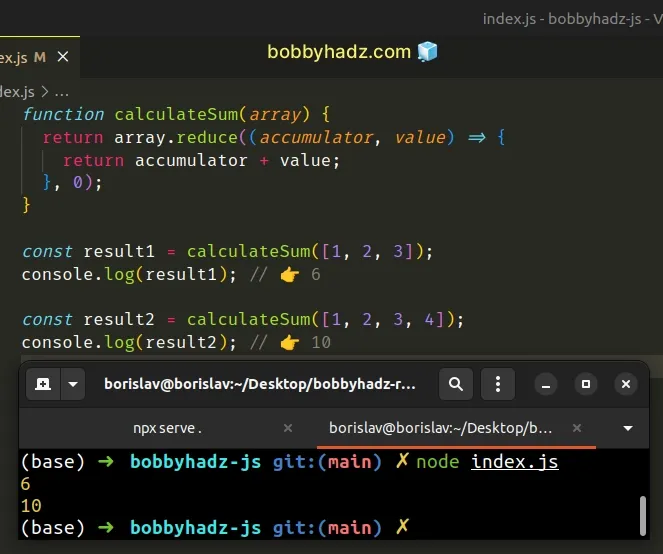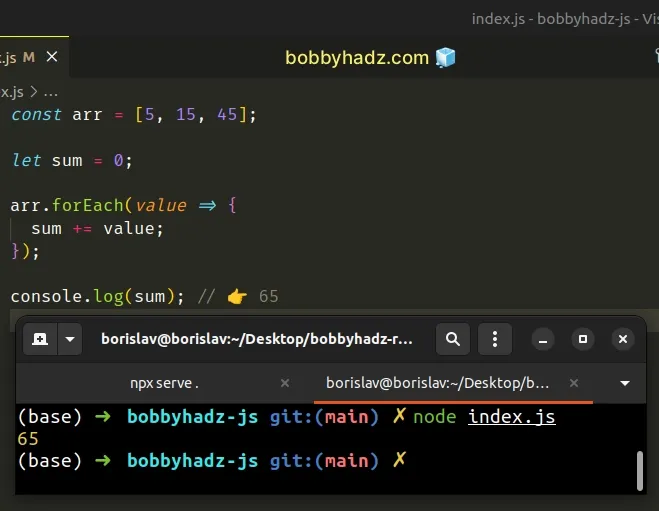# Get the Sum of an Array of Numbers in JavaScriptLast updated: Dec 26, 2022
5 min## #Get the Sum of an Array of Numbers in JavaScript

To get the sum of an array of numbers:

1. Use the `Array.reduce()` method to iterate over the array.
2. Set the initial value in the `reduce` method to `0`.
3. On each iteration, return the sum of the accumulated value and the current number.
index.js
```Copied!```const arr = [5, 15, 45];

const sum = arr.reduce((accumulator, value) => {
return accumulator + value;
}, 0);

console.log(sum); // 👉️ 65
``````The function we passed to the Array.reduce method gets called for each element in the array.

We initialized the `accumulator` variable to `0` because that's what we passed as the second argument to the `reduce()` method.

On each iteration, we return the sum of the `accumulator` and the current array element.

After the last iteration, the `sum` variable stores the sum of the numbers in the array.

## #Creating a reusable function

If you have to calculate the sum of arrays often, define a reusable function.

index.js
```Copied!```function calculateSum(array) {
return array.reduce((accumulator, value) => {
return accumulator + value;
}, 0);
}

const result1 = calculateSum([1, 2, 3]);
console.log(result1); // 👉️ 6

const result2 = calculateSum([1, 2, 3, 4]);
console.log(result2); // 👉️ 10
``````The `calculateSum()` function takes an array as a parameter and calculates the sum of the numbers in the array.

You can also shorten the function we passed to the `reduce()` method by using an implicit return statement.

index.js
```Copied!```function calculateSum(array) {
return array.reduce((accumulator, value) => accumulator + value, 0);
}

const result1 = calculateSum([1, 2, 3]);
console.log(result1); // 👉️ 6

const result2 = calculateSum([1, 2, 3, 4]);
console.log(result2); // 👉️ 10
``````

The arrow function in the example uses an implicit return.

An alternative and perhaps simpler approach is to use a `for...of` loop.

## #Get the Sum of an Array of Numbers using a `for...of` loop

This is a three-step process:

1. Declare a `sum` variable and initialize it to `0`.
2. Use the `for...of` loop to iterate over the array.
3. On each iteration, reassign the `sum` variable to its current value plus the value of the current element.
index.js
```Copied!```const arr = [5, 15, 45];

let sum = 0;

for (const value of arr) {
sum += value;
}

console.log(sum); // 👉️ 65
``````If you need to handle non-numeric values, use the `typeof` operator.

index.js
```Copied!```// 👇️ array contains non-numeric values
const arr = ['a', 5, 'b', 15, 'c', 45];

let sum = 0;

for (const value of arr) {
if (typeof value === 'number') {
sum += value;
}
}

console.log(sum); // 👉️ 65
``````

The array in the code sample also contains non-numeric values, so we used the `typeof` operator to check if each value is a number before adding it to the total.

The for...of statement is used to loop over iterable objects like arrays, strings, `Map`, `Set` and `NodeList` objects and `generators`.

Notice that we declared the `sum` variable using the `let` keyword. Had we declared the variable using `const`, we wouldn't be able to reassign it.

On each iteration, we reassign the `sum` variable to its current value plus the value of the current element.

If you have to do this often, extract the logic into a reusable function.

index.js
```Copied!```function calculateSum(array) {
let total = 0;

for (const value of array) {
total += value;
}

}

const result1 = calculateSum([1, 2, 3]);
console.log(result1); // 👉️ 6

const result2 = calculateSum([1, 2, 3, 4]);
console.log(result2); // 👉️ 10
``````

The `calculateSum()` function takes an array as a parameter, calculates the sum of its values and returns the result.

Alternatively, you can use `Array.forEach()`.

## #Get the Sum of an Array of Numbers using `Array.forEach()`

This is a three-step process:

1. Declare a new `sum` variable and initialize it to `0`.
2. Use the `Array.forEach()` method to iterate over the array.
3. On each iteration, reassign the value of the sum variable to its current value plus the array element.
index.js
```Copied!```const arr = [5, 15, 45];

let sum = 0;

arr.forEach(value => {
sum += value;
});

console.log(sum); // 👉️ 65
``````The function we passed to the Array.forEach method gets called with each element in the array.

On each iteration, we reassign the value of the `sum` variable to its current value plus the value of the current array element.

The `sum` variable stores the sum of the values in the array after the last iteration.

If you have to calculate the sum of an array's elements often, define a reusable function.

index.js
```Copied!```function calculateSum(array) {
let total = 0;

array.forEach(value => {
total += value;
});

}

const result1 = calculateSum([1, 2, 3]);
console.log(result1); // 👉️ 6

const result2 = calculateSum([1, 2, 3, 4]);
console.log(result2); // 👉️ 10
``````

The `calculateSum()` function takes an array as a parameter, calculates the sum of the array's elements and returns the result.

Alternatively, you can use a basic `for` loop.

## #Get the Sum of an Array of Numbers using a `for` loop

This is a three-step process:

1. Declare a new `sum` variable and initialize it to `0`.
2. Use a `for` loop to iterate over the array.
3. Reassign the value of the `sum` variable to its current value plus the current array element.
index.js
```Copied!```const arr = [5, 15, 45];

let sum = 0;

for (let index = 0; index < arr.length; index++) {
sum += arr[index];
}

console.log(sum); // 👉️ 65
``````We used a basic `for` loop to iterate over the array.

On each iteration, we use the index to access the current array element and reassign the `sum` variable.

Which approach you pick is a matter of personal preference. I'd use the `Array.reduce()` method or a `for...of` loop as both options are quite direct and intuitive.

## #Get the Sum of an Array of Numbers using a `while` loop

You can also use a `while` loop to get the sum of an array of numbers.

index.js
```Copied!```const arr = [5, 15, 45];

let sum = 0;
let i = -1;

while (++i < arr.length) {
sum += arr[i];
}

console.log(sum); // 👉️ 65
``````We initialized the `sum` and `i` variables using the `let` keyword.

Using `let` is important because variables declared using `const` cannot be reassigned.

On each iteration of the `while` loop, we increment the `i` variable and add the current array element to the total.

## #Get the Sum of an Array of Numbers using `lodash`

If you use the `lodash` library, you can also use the `lodash.sum()` method to get the sum of an array of numbers.

index.js
```Copied!```import _ from 'lodash';

console.log(_.sum([1, 2, 3])); // 👉️ 6

console.log(_.sum([10, 20, 30])); // 👉️ 60
``````If you need to install `lodash`, run the following command.

shell
```Copied!```# 👇️ initialize a package.json file if you don't have one
npm init -y

npm install lodash
``````

The lodash.sum method takes an array as a parameter and computes the sum of the values in the array.